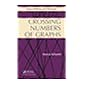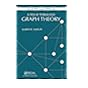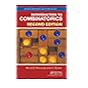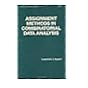Normal view MARC view ISBD view

# Introduction to combinatorics

Material type:BookPublisher: London Taylor and Francis 2017Edition: 2nd.Description: xix, 423 p.ISBN: 9781138582620.DDC classification: 511​.6 Summary: What Is Combinatorics Anyway? Broadly speaking, combinatorics is the branch of mathematics dealing with different ways of selecting objects from a set or arranging objects. It tries to answer two major kinds of questions, namely, counting questions: how many ways can a selection or arrangement be chosen with a particular set of properties; and structural questions: does there exist a selection or arrangement of objects with a particular set of properties? The authors have presented a text for students at all levels of preparation. For some, this will be the first course where the students see several real proofs. Others will have a good background in linear algebra, will have completed the calculus stream, and will have started abstract algebra. The text starts by briefly discussing several examples of typical combinatorial problems to give the reader a better idea of what the subject covers. The next chapters explore enumerative ideas and also probability. It then moves on to enumerative functions and the relations between them, and generating functions and recurrences., Important families of functions, or numbers and then theorems are presented. Brief introductions to computer algebra and group theory come next. Structures of particular interest in combinatorics: posets, graphs, codes, Latin squares, and experimental designs follow. The authors conclude with further discussion of the interaction between linear algebra and combinatorics. (https://www.crcpress.com/Introduction-to-Combinatorics/Wallis-George/p/book/9781498777605)
Tags from this library: No tags from this library for this title.
Item type Current location Item location Collection Call number Status Date due Barcode
Books Vikram Sarabhai Library
General Stacks
Slot 1358 (0 Floor, East Wing) Non-fiction 511​.6 W2I6 (Browse shelf) Available 198839
##### Browsing Vikram Sarabhai Library Shelves , Shelving location: General Stacks , Collection code: Non-fiction Close shelf browser511.5 R4A2 Advanced graph theory and combinatorics 511.5 S2C7 Crossing numbers of graphs 511.5 S2T6 A tour through graph theory 511​.6 W2I6 Introduction to combinatorics 511.6 A7T7 The traveling salesman problem: a computational study 511.6 B2A2 Additive combinatorics: a menu of research problems 511.6 H8A8 Assignment methods in combinatorial data analysis

Introduction
Some Combinatorial Examples
Sets, Relations and Proof Techniques
Two Principles of Enumeration
Graphs
Systems of Distinct Representatives

Fundamentals of Enumeration
Permutations and Combinations
Applications of P(n, k) and (n k)
Permutations and Combinations of Multisets
Applications and Subtle Errors
Algorithms

Probability
Introduction
Some Definitions and Easy Examples
Events and Probabilities
Three Interesting Examples
Probability Models
Bernoulli Trials
The Probabilities in Poker

The Pigeonhole Principle and Ramsey’s Theorem
The Pigeonhole Principle
Applications of the Pigeonhole Principle
Ramsey’s Theorem — the Graphical Case
Ramsey Multiplicity
Sum-Free Sets
Bounds on Ramsey Numbers
The General Form of Ramsey’s Theorem

The Principle of Inclusion and Exclusion
Unions of Events
The Principle
Combinations with Limited Repetitions
Derangements
Generating Functions and Recurrence Relations
Generating Functions
Recurrence Relations
From Generating Function to Recurrence
Exponential Generating Functions

Catalan, Bell and Stirling Numbers
Introduction
Catalan Numbers
Stirling Numbers of the Second Kind
Bell Numbers
Stirling Numbers of the First Kind
Computer Algebra and Other Electronic Systems

Symmetries and the P´olya-Redfield Method
Introduction
Basics of Groups
Permutations and Colorings
An Important Counting Theorem
P´olya and Redfield’s Theorem
Partially-Ordered Sets
Introduction
Examples and Definitions
Bounds and lattices
Isomorphism and Cartesian products
Extremal set theory: Sperner’s and Dilworth’s theorems

Introduction to Graph Theory
Degrees
Paths and Cycles in Graphs
Maps and Graph Coloring

Further Graph Theory
Euler Walks and Circuits
Application of Euler Circuits to Mazes
Hamilton Cycles
Trees
Spanning Trees

Coding Theory
Errors; Noise
The Venn Diagram Code
Binary Codes; Weight; Distance
Linear Codes
Hamming Codes
Codes and the Hat Problem
Variable-Length Codes and Data Compression

Latin Squares
Introduction
Orthogonality
Idempotent Latin Squares
Partial Latin Squares and Subsquares
Applications

Balanced Incomplete Block Designs
Design Parameters
Fisher’s Inequality
Symmetric Balanced Incomplete Block Designs
New Designs from Old
Difference Methods

Linear Algebra Methods in Combinatorics
Recurrences Revisited
State Graphs and the Transfer Matrix Method
Kasteleyn’s Permanent Method

Appendix 1: Sets; Proof Techniques7
Appendix 2: Matrices and Vectors
Appendix 3: Some Combinatorial People

What Is Combinatorics Anyway?
Broadly speaking, combinatorics is the branch of mathematics dealing with different ways of selecting objects from a set or arranging objects. It tries to answer two major kinds of questions, namely, counting questions: how many ways can a selection or arrangement be chosen with a particular set of properties; and structural questions: does there exist a selection or arrangement of objects with a particular set of properties?
The authors have presented a text for students at all levels of preparation.
For some, this will be the first course where the students see several real proofs. Others will have a good background in linear algebra, will have completed the calculus stream, and will have started abstract algebra.
The text starts by briefly discussing several examples of typical combinatorial problems to give the reader a better idea of what the subject covers. The next chapters explore enumerative ideas and also probability. It then moves on to enumerative functions and the relations between them, and generating functions and recurrences., Important families of functions, or numbers and then theorems are presented. Brief introductions to computer algebra and group theory come next. Structures of particular
interest in combinatorics: posets, graphs, codes, Latin squares, and experimental designs follow. The authors conclude with further discussion of the interaction between linear algebra and combinatorics.

(https://www.crcpress.com/Introduction-to-Combinatorics/Wallis-George/p/book/9781498777605)

There are no comments for this item.